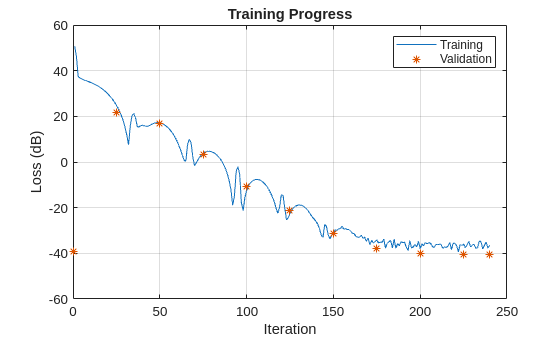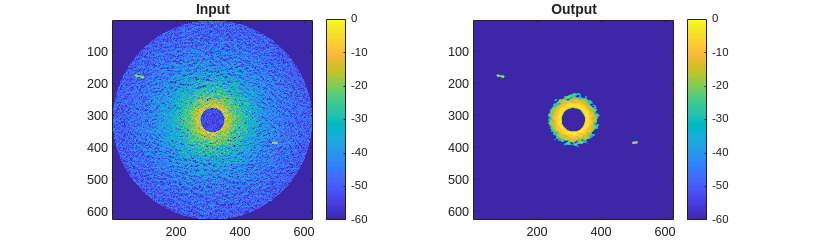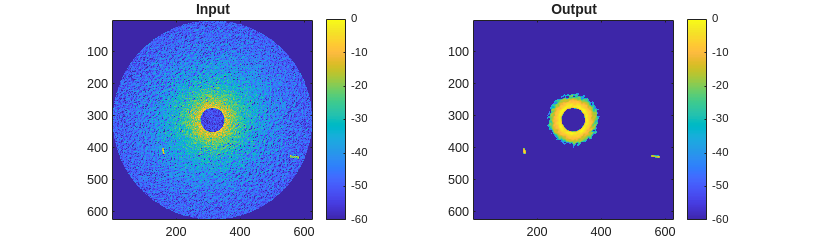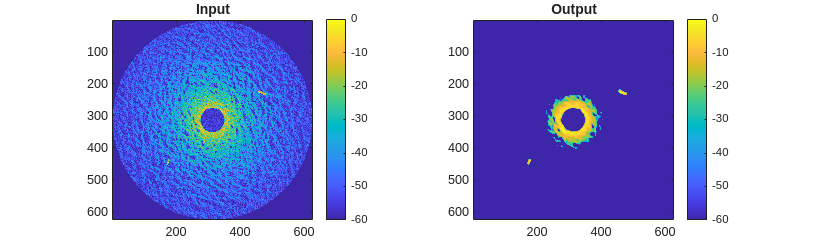# Maritime Clutter Removal with Neural Networks

This example shows how to train and evaluate a convolutional neural network to remove clutter returns from maritime radar PPI images using the Deep Learning Toolbox™. The Deep Learning Toolbox provides a framework for designing and implementing deep neural networks with algorithms, pretrained models, and apps. The Simulate a Maritime Radar PPI example demonstrates how to use Radar Toolbox™ to create these images.

### The Dataset

The dataset contains 84 pairs of synthetic radar images. Each pair consists of an input image, which has both sea clutter and extended target returns, and a desired response image, which includes only the target returns. The images were created using a `radarScenario` simulation with a `radarTransceiver` and a rotating uniform linear array (ULA). Each image contains two nonoverlapping extended targets with one representing a small container ship and the other representing a larger container ship. The ships were modeled by a set of point scatterers on the surface of a cuboid.

The following parameters are fixed from image to image:

• Frequency (10 GHz)

• Pulse length (80 ns)

• Range resolution (7.5 m)

• PRF (1 kHz)

• Azimuth beamwidth (0.28 deg)

• Height (55 m)

• Rotation rate (50 RPM)

• Target parameters

• Small target dimensions (120-by-18-by-22 m)

• Large target dimensions (200-by-32-by-58 m)

The following parameters are randomized from image to image:

• Surface parameters

• Wind speed (7 to 17 m/s)

• Wind direction (0 to 180 deg)

• Target parameters

• Target position (anywhere on the surface)

• Target heading (0 to 360 deg)

• Target speed (4 to 19 m/s)

• Small target RCS (8 to 16 m${}^{2}$)

• Large target RCS (14 to 26 m${}^{2}$)

This variation ensures that a network trained on this data will be applicable to a fairly wide range of target profiles and sea states for this radar configuration. For more information on sea states, see the Maritime Radar Sea Clutter Modeling example.

```dataURL = 'https://ssd.mathworks.com/supportfiles/radar/data/MaritimeRadarPPI.zip'; unzip(dataURL)```

Load the image data and pretrained network into a struct called `imdata`.

`imdata = load('MaritimeRadarPPI.mat');`

### Prepare the Data

You can use the pretrained network to run the example without having to wait for training. To perform the training steps, set the `doTrain` variable to `true` by selecting the check box below.

```doTrain =false; if ~doTrain load PPIDeclutterNetwork.mat end```

Image sets 1-70 are used for training and 71-80 for validation. The last 4 images will be used for evaluation of the network.

Format the data as a 4D array for use with the network trainer and training options. The first two dimensions are considered spatial dimensions. The third dimension is for channels (such as color channels). The separate image samples are arranged along the 4th dimension. The cluttered inputs are simply referred to as images, and the desired output is known as the response. Single precision is used since that is native to the neural network trainer.

```imgs = zeros(626,626,1,84,'single'); resps = zeros(626,626,1,84,'single'); for ind = 1:84 imgs(:,:,1,ind) = imdata.(sprintf('img%d',ind)); resps(:,:,1,ind) = imdata.(sprintf('resp%d',ind)); end```

After formatting, clear the loaded data struct to save RAM.

`clearvars imdata`

### Network Architecture

A network is defined by a sequence of layer objects, including an input and output layer. An `imageInputLayer` is used as the input layer so that the images may be used without any reformatting. A `regressionLayer` is used for the output to evaluate a simple mean-squared-error (MSE) loss function. A cascade of 2D convolution layers with normalizations and nonlinear activations is used for the hidden layers.

Start by creating the input layer. Specify the spatial size of the input images.

`layers = imageInputLayer([626 626]);`

Add 3 sets of convolution+normalization+activation. Each convolution layer consists of a set of spatial filters. The `batchNormalizationLayer` biases and scales each mini batch to improve numerical robustness and speed up training. The `leakyReluLayer` is a nonlinear activation layer that scales values below 0 while leaving values greater than 0 unmodified. The `tanhLayer` is a sigmoidal activation layer made from a hyperbolic tangent function that outputs values between -1 and 1.

Care must be taken to ensure the spatial and channel dimensions are consistent from layer to layer and that the size and number of channels of the output from the last layer matches the size and number of channels of the desired response images. Set the `Padding` property of the convolution layers to `'same'` so that the filtering process does not change the spatial size of the images.

The architecture can be summarized as follows:

• 1x 5-by-5 convolution

• Batch normalization

• Leaky ReLU with 0.2 scaling

• 4x 6-by-6 convolution

• Batch normalization

• Leaky ReLU with 0.2 scaling

• 1x 5-by-5 convolution

• Batch normalization

• Leaky ReLU with 0.2 scaling

```layers(end+1) = convolution2dLayer([5 5],1,'NumChannels',1,'Padding','same'); layers(end+1) = batchNormalizationLayer; layers(end+1) = leakyReluLayer(0.2); layers(end+1) = convolution2dLayer([6 6],4,'NumChannels',1,'Padding','same'); layers(end+1) = batchNormalizationLayer; layers(end+1) = leakyReluLayer(0.2); layers(end+1) = convolution2dLayer([5 5],1,'NumChannels',4,'Padding','same'); layers(end+1) = batchNormalizationLayer; layers(end+1) = leakyReluLayer(0.2);```

Note that the number of channels of each convolution layer must match the number of filters used in the previous convolution layer.

Finally, add the output layer, which is a simple regression layer.

`layers(end+1) = regressionLayer;`

### Train the Network

Use the `trainingOptions` function to configure exactly how the network is trained. In addition to specifying the training method to use, this provides control over things like learn-rate scheduling and the size of mini batches. The `trainingOptions` can also be used to specify a validation data set, which is used to determine the running performance. Since the performance of a network may not improve monotonically with iterations, this also provides a way to return the network at whichever iteration yielded the lowest validation error.

Set the random seed for repeatability.

`rng default`

Define the IDs of the sets to use for training and for validation.

```trainSet = 1:70; valSet = 71:80;```

Now create the `trainingOptions`. Use the adaptive moment estimation (Adam) solver. Train for a maximum of 80 epochs with a mini batch size of 20. Set the initial learn rate to 0.1. The validation set is specified with a 1-by-2 cell array containing the validation image and response arrays. Set the `ValidationFrequency` to 25 to evaluate the loss for the validation set every 25 iterations. Specify `OutputNetwork` as `'best-validation-loss'` to return the network at the iteration which had the least validation loss. Set `Verbose` to true to print the training progress.

```opts = trainingOptions("adam", ... MaxEpochs=80, ... MiniBatchSize=20, ... Shuffle="every-epoch", ... InitialLearnRate=0.1, ... ValidationData={imgs(:,:,:,valSet),resps(:,:,:,valSet)}, ... ValidationFrequency=25, ... OutputNetwork='best-validation-loss', ... Verbose=true);```

Training is initiated with the `trainNetwork` method. Input the 4D training image and response arrays, the vector of network layers, and the training options. This will only run if the `doTrain` flag is set to `true`.

A compatible GPU is used by default if available. Using a GPU requires Parallel Computing Toolbox™ and a supported GPU device. For information on supported devices, see GPU Computing Requirements (Parallel Computing Toolbox). Otherwise, `trainNetwork` uses the CPU.

```if doTrain [net,info] = trainNetwork(imgs(:,:,:,trainSet),resps(:,:,:,trainSet),layers,opts); end```

Use the provided helper function to plot the training and validation loss on a log scale.

`helperPlotTrainingProgress(info)`The training and validation loss decreased steadily until an error floor was hit at around interation 200.

### Evaluate the Network

Now that the network has been trained, use the last 4 images to evaluate the network.

`evalSet = 81:84;`

Use the provided helper function to plot the input images alongside the responses output by the network. The results are normalized and pixels below -60 dB are clipped for easier comparison.

`helperPlotEvalResults(imgs(:,:,:,evalSet),net);`The network completely removes the sea clutter below a certain threshold of returned power while retaining the target signals with only a small dilation effect due to the size of the convolution filters used. The remaining high-power clutter near the center of the images could be removed by a spatially-aware layer, such as a fully-connected layer, or by preprocessing the original images to remove the range-dependent losses.

### Conclusion

In this example, you saw how to train and evaluate a cascaded convolutional neural network on PPI images to remove sea clutter while retaining target returns. You saw how to configure the input and output layers, the hidden convolution, normalization, and activation layers, and the training options.

### Reference

 Vicen-Bueno, Raúl, Rubén Carrasco-Álvarez, Manuel Rosa-Zurera, and José Carlos Nieto-Borge. “Sea Clutter Reduction and Target Enhancement by Neural Networks in a Marine Radar System.” Sensors (Basel, Switzerland) 9, no. 3 (March 16, 2009): 1913–36.

### Supporting Functions

#### helperPlotTrainingProgress

```function helperPlotTrainingProgress(info) % Plot training progress plot(10*log10(info.TrainingLoss)) hold on plot(10*log10(info.ValidationLoss),'*') hold off grid on legend('Training','Validation') title('Training Progress') xlabel('Iteration') ylabel('Loss (dB)') end```

#### helperPlotEvalResults

```function helperPlotEvalResults(imgs,net) % Plot original images alongside desired and actual responses for ind = 1:size(imgs,4) resp_act = predict(net,imgs(:,:,1,ind)); resp_act(resp_act<0) = 0; resp_act = resp_act/max(resp_act(:)); fh = figure; subplot(1,2,1) im = imgs(:,:,1,ind); im = im/max(im(:)); imagesc(20*log10(im)) clim([-60 0]) colorbar axis equal axis tight title('Input') subplot(1,2,2) imagesc(20*log10(resp_act)) clim([-60 0]) colorbar axis equal axis tight title('Output') fh.Position = fh.Position + [0 0 560 0]; end end```